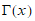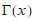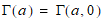# NMath User's Guide

39.2 Gamma Function (.NET, C#, CSharp, VB, Visual Basic, F#)

The static GammaLn() method on class StatsFunctions evaluates the log of the gamma functionat a value x. The gamma function is an extension of the factorial function to complex and real number arguments.

The "complete" gamma functioncan be generalized to the incomplete gamma function, such that. The "lower" incomplete gamma function is given by:IncompleteGamma() returns the value of the lower regularized incomplete gamma function.

Top

Top